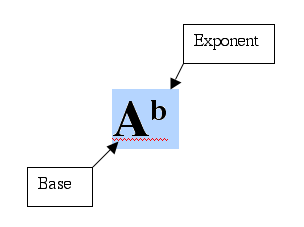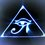# Types of ExponentsTypes of Exponents and Types of Numbers

Before we get into doing operations with exponents lets make sure we understand the different types of exponents. There are many types of exponents as there are many types of numbers. I will list the basic ones that will provide you with the understanding to get through most problems. Keep in mind that negative and rational exponents can be combined.

Counting Numbers: An exponent that is number you can count like if you were counting sheep. $(1,2,3)$

Zero exponents: An exponent that is zero. $(0)$

Negative Integers: An exponent that is a negative number but is not a fraction. $(-1,-2,-3)$

Rational Numbers: An exponent that is a fraction $(\frac{1}{2}, \frac{3}{4}, \frac{5}{6} )$

Counting Number Exponents

Lets start off by explaining the counting number exponents $(1,2,3)$.

If you have an base $a$ raised to the exponent $b$ such that $a^{b}$, then your are multiplying $a$ by $1$, $b$ times.

$a^{1}=1 \times a$

$a^{2}=1 \times a \times a$

$a^{5}= 1\times a \times a \times a \times a \times a$

Zero Exponents

What if you have an exponent that is zero. Well, if you just read the section on counting numbers and asked why there was a one out front, it will probably make sense now. If you have a base $a$ raised to the exponent $0$, then you multiply $a$ by $1$ zero times, leaving you with $1$. Any number to the exponent zero is just $1$.

$a^{0}=1$

Negative Integer Exponents

When You have a base $a$ raised to a negative integer exponent $b$ such that $a^{-b}$ you divide $1$ by $a$, $b$ times.

$a^{-1}= 1 \div a$

$a^{-1}= \frac{1}{a}$

$a^{-2}= 1 \div a \div a$

$a^{-2}= \frac{1}{a^{2}}$

$a^{-5}= 1 \div a \div a \div a \div a \div a$

$a^{-5}= \frac{1}{a^{5}}$

Rational Exponents

Rational, or fractional exponents turn into radicals or roots. If there is an expression $a^{\frac{b}{c}}$ then you find the $c$ root of $a$ and then raise it to the exponent $b$. $c$ is reffered to as the index which tells you which root you are taking.

The general rule is that $a^{\frac{b}{c}}=\sqrt[c]{a^{b}}$

example

$64^{\frac{2}{3}}$

$= \sqrt{64^{2}}$ OR $(\sqrt{64})^{2}$

$= \sqrt{4096}$ OR $4^{2}$

$=16$Note by Brody Acquilano
6 years, 3 months ago

This discussion board is a place to discuss our Daily Challenges and the math and science related to those challenges. Explanations are more than just a solution — they should explain the steps and thinking strategies that you used to obtain the solution. Comments should further the discussion of math and science.

When posting on Brilliant:

• Use the emojis to react to an explanation, whether you're congratulating a job well done , or just really confused .
• Ask specific questions about the challenge or the steps in somebody's explanation. Well-posed questions can add a lot to the discussion, but posting "I don't understand!" doesn't help anyone.
• Try to contribute something new to the discussion, whether it is an extension, generalization or other idea related to the challenge.

MarkdownAppears as
*italics* or _italics_ italics
**bold** or __bold__ bold
- bulleted- list
• bulleted
• list
1. numbered2. list
1. numbered
2. list
Note: you must add a full line of space before and after lists for them to show up correctly
paragraph 1paragraph 2

paragraph 1

paragraph 2

[example link](https://brilliant.org)example link
> This is a quote
This is a quote
    # I indented these lines
# 4 spaces, and now they show
# up as a code block.

print "hello world"
# I indented these lines
# 4 spaces, and now they show
# up as a code block.

print "hello world"
MathAppears as
Remember to wrap math in $$ ... $$ or $ ... $ to ensure proper formatting.
2 \times 3 $2 \times 3$
2^{34} $2^{34}$
a_{i-1} $a_{i-1}$
\frac{2}{3} $\frac{2}{3}$
\sqrt{2} $\sqrt{2}$
\sum_{i=1}^3 $\sum_{i=1}^3$
\sin \theta $\sin \theta$
\boxed{123} $\boxed{123}$

Sort by: